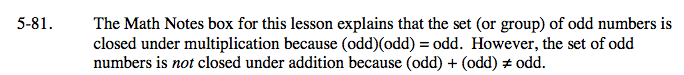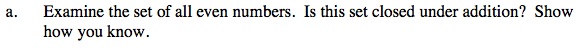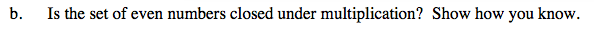### Home > CAAC > Chapter 5 > Lesson 5.2.2 > Problem5-81

5-81.Even numbers are closed under addition, because the sum of two even numbers is also even.

For example,
4 + 4 = 8
10 + 10 = 20
36 + 36 = 72What happens if you mulitply two even numbers together?

For example,
(4)(4) = 16
(10)(10) = 100
(36)(36) = 1296